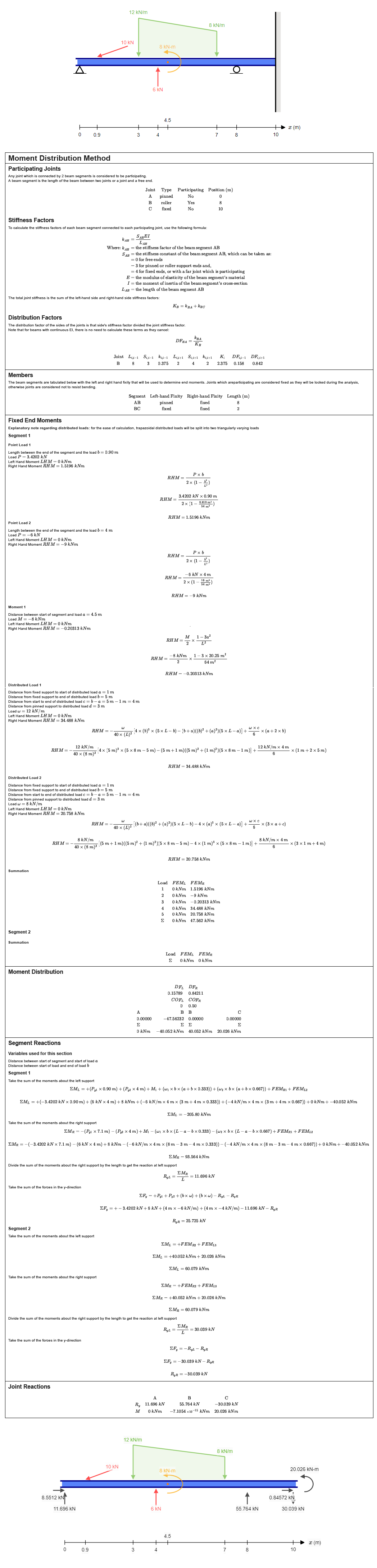# Moment Distribution Method Hand Calculations for Indeterminate Beams

At SkyCiv we believe education is important so we're committed to helping the next generation of engineers learn structural engineering. As part of this mission we've been developing hand calculation reports that open the black box of structural engineering software, exposing it to the curious minds of our users. Engineers like to know the how and why something is the way it is, and often the best way to learn this is by trying to work it out yourself and comparing your solution to one which is complete and correct. We balance the readability of our reports with the level of detail required to back check the calculations, step by step. This is fundamental to engineering practice and we will continue to provide users with tools that add value to their education, work and lives.

We're driven by what you want: one of the most requested features from our users was hand calculations for indeterminate beams. We are proud to announce this feature is now live: current Student, Basic and Professional users can try it now. For indeterminate beams without hinges, the vertical and moment reactions are solved using the Moment Distribution Method.

The MDM Hand Calculation module, will easily show the hand calculations for a beam such as the following:Existing users will be familiar with accessing hand calculations for statically determinate structures in Beam however, if you're new to SkyCiv or haven't tried found hand calculations yet, our documentation can guide you through the process.

## Moment Distribution Method

Moment Distribution Method (MDM), invented by Hardy Cross, is extremely powerful in it’s simplicity and has been used extensively since it was first published by the ASCE journal in 1930. Part of its simplicity lies in the way that the bending moment diagram can be drawn graphically using superposition without needing to calculate the discontinuity equations for each segment. Complex structures can be solved relatively easily compared with other methods such as FEM, the Virtual Displacement Method, or solving discontinuity equations. MDM can be used on a variety of 2D structures like beams and frames.

Hardy Cross was a structural engineer in a period of time when hand calculation was an indispensable exercise for any structural engineer. After repeating the process of calculating structures countless times he recognized that he was essentially completing the same calculations over and over again. This led Cross to explore a theory that complex structures were actually combinations of simple structures and, these simple structures could be assembled to create the complex structure with the same analytical solution. Simple structures are easy to solve, formulas were easy enough to derive even if they were indeterminate, thanks to superposition and the Virtual Force Method. Cross created tables of these simple structures and went about testing his theory in various ways.

## Fixed End Moment Equations

Moment Distribution Method uses tables of common loading cases to look up the equations for the moments at the ends of each segment. These tables can be found in our documentation for calculating bending moment diagrams and not only form the basis on which the MDM is used, but also is a great reference for simple bending moment calculations. A simple way to look at it is, the MDM breaks the beam into segments, and uses these simple equations to calculate the BMD.

## Alternative Methods

Calculating the bending moment diagram can be achieve in other ways, like using discontinuous equations which we wrote about previously here.

## Sample Report